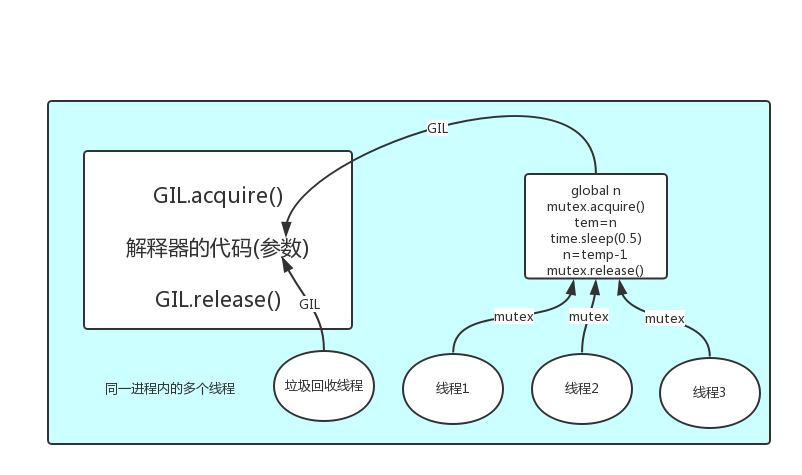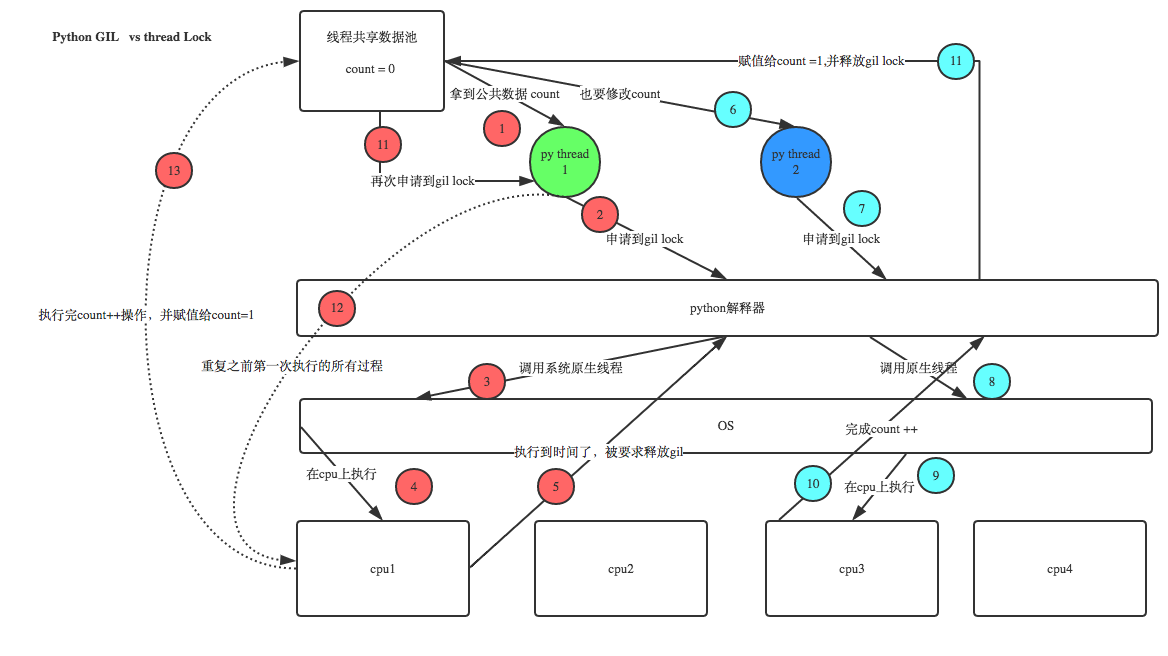# 全局解释器锁GIL &amp; 线程锁

## 1.GIL锁(Global Interpreter Lock)

Python代码的执行由Python虚拟机来控制。Python在设计之初就考虑到要在主循环中，同时只有一个线程在执行。虽然 Python 解释器中可以“运行”多个线程，但在任意时刻只有一个线程在解释器中运行。

对Python虚拟机的访问由全局解释器锁来控制，正是这个锁能保证同一时刻只有一个线程在运行。

GIL本质就是一把互斥锁，既然是互斥锁，所有互斥锁的本质都一样，都是将并发运行变成串行，以此来控制同一时间内共享数据只能被一个任务所修改，进而保证数据安全。

### python程序运行的过程

1.在内存中加载生成一个进程的内存空间

2.这个内存空间中有有解释器的代码和你要执行进程的代码

3.进程中又有多个线程

4.由于python是一门解释型语言, CPU无法识别python的代码, 所以必须经过解释器来对代码进行编译成二进制的代码

5.但是由于CPython解释器的特性,有一个GIL锁,同一时刻只能有一个线程能进入解释器进行编译

6.当一个线程运行了指定数量的字节码指令或者遇到I/O操作时, GIL锁才会被释放, 后面的线程再去抢GIL锁重复前面的操作

7.所以对于同一个进程中的多个线程是无法同时使用多个CPU的## 2.同步锁

当同时异步提交多个线程, 这些线程都有相同的数据修改操作, 且在数据修改操作之前有I/O操作或者网络延时, 这个时候可能多个进程拿到的数据是相同的, 然后修改后的数据也是相同的, 这样就会造成数据混乱问题. 这个时候就能用到同步锁, 在拿数据前加个锁,修改完之后再释放, 这样就不会有数据混乱的问题.``````from threading import Threadimport os,timedef work():    global n    temp=n    time.sleep(0.1)    n=temp-1if __name__ == '__main__':    n=100    l=[]    for i in range(100):        p=Thread(target=work)        l.append        p.start()    for p in l:        p.join()    print #结果可能为99
``````

``````from threading import Thread,Lockimport os,timedef work():    global n    lock.acquire()    temp=n    time.sleep(0.1)    n=temp-1    lock.release()if __name__ == '__main__':    lock=Lock()    n=100    l=[]    for i in range(100):        p=Thread(target=work)        l.append        p.start()    for p in l:        p.join()    print #结果肯定为0，由原来的并发执行变成串行，牺牲了执行效率保证了数据安全
``````

### 死锁和递归锁``````import timefrom threading import Thread, Lock, RLockdef func1(lockA,lockB):    lockA.acquire()    time.sleep(1)    print("锁A")    lockB.acquire()    print("锁B")    lockB.release()    lockA.release()def func2(lockA,lockB):    lockB.acquire()    print("锁A")    lockA.acquire()    print("锁B")    lockA.release()    lockB.release()if __name__ == '__main__':    lockA = Lock()    lockB = Lock()    t1 = Thread(target=func1, args=(lockA,lockB ))    t1.start()    t2 = Thread(target=func2, args=(lockA, lockB))    t2.start()
````````````import timefrom threading import Thread, Lock, RLockdef func1(lockA,lockB):    lockA.acquire()    time.sleep(1)    print("锁A")    lockB.acquire()    print("锁B")    lockB.release()    lockA.release()def func2(lockA,lockB):    lockB.acquire()    print("锁A")    lockA.acquire()    print("锁B")    lockA.release()    lockB.release()if __name__ == '__main__':    lockA = lockB = RLock()    t1 = Thread(target=func1, args=(lockA,lockB ))    t1.start()    t2 = Thread(target=func2, args=(lockA, lockB))    t2.start()
``````

• 线程概论
• python的并发编程之多线程
• Python GIL(Global Interpreter Lock)

## 3.GIL锁与同步锁LOCK

GIL是解释器级别的, 保护的是解释器级别的数据, 比如垃圾回收的数据.

Lock是保护用户自己开发的应用程序的数据.### 互斥锁和join的区别

start后立即join: 任务内的所有代码都是串行执行的, 而加锁, 只是加锁的部分即修改共享数据的部分是串行的.

1、何为线程

2、线程和进程的区别

``````1.Threads share the address space of the process that created it; processes have their own address space.
2.Threads have direct access to the data segment of its process; processes have their own copy of the data segment of the parent process.
3.Threads can directly communicate with other threads of its process; processes must use interprocess communication to communicate with sibling processes.
4.New threads are easily created; new processes require duplication of the parent process.
5.Threads can exercise considerable control over threads of the same process; processes can only exercise control over child processes.
6.Changes to the main thread (cancellation, priority change, etc.) may affect the behavior of the other threads of the process; changes to the parent process does not affect child processes.
``````

``````1、线程共享创建它的进程的地址空间;进程有自己的地址空间。
2、线程可以直接访问其进程的数据段;进程有它们自己的父进程数据段的副本。
3、线程可以直接与进程的其他线程通信;进程必须使用进程间通信来与兄弟进程通信。
4、新线程很容易创建;新进程需要复制父进程。
5、线程可以对同一进程的线程进行相当大的控制;进程只能对子进程执行控制。
6、对主线程的更改(取消、优先级更改等)可能会影响该进程的其他线程的行为;对父进程的更改不会影响子进程。
``````

3、多线程的优点

1）多线程共享一个进程的地址空间（资源）

2） 线程比进程更轻量级，线程比进程更容易创建可撤销，在许多操作系统中，创建一个线程比创建一个进程要快10-100倍，在有大量线程需要动态和快速修改时，这一特性很有用

3） 若多个线程都是cpu密集型的，那么并不能获得性能上的增强，但是如果存在大量的计算和大量的I/O处理，拥有多个线程允许这些活动彼此重叠运行，从而会加快程序执行的速度。

4） 在多cpu系统中，为了最大限度的利用多核，可以开启多个线程，比开进程开销要小的多。（这一条并不适用于python）

30、进程的基础理论，并发（multiprocessing模块）：

2、开启线程的两种方式（和进程一模一样）

1）方式一：

``````from threading import Thread
#from  multiprocessing  import  Process
import os
def talk():
print('%s is running' %os.getpid())

if __name__ == '__main__':
# t=Process(target=talk)
t.start()
print('主',os.getpid())
``````

2）方式二：

``````#开启线程
import os
def __init__(self,name):
super().__init__()
self.name=name
def run(self):
print('pid:%s name:[%s]is running' %(os.getpid(),self.name))

if __name__ == '__main__':
t.start()
print('主T',os.getpid())

#开启进程
from multiprocessing import Process
import os
class MyProcess(Process):
def __init__(self,name):
super().__init__()
self.name=name
def run(self):
print('pid:%s name:[%s]is running' % (os.getpid(), self.name))
if __name__ == '__main__':
t=MyProcess('lll')
t.start()
print('主P',os.getpid())
``````

3、在一个进程下开启多个线程与在一个进程下开启多个子进程的区别

1）比较速度：（看看hello和主线程/主进程的打印速度）

``````from threading import Thread
from multiprocessing import Process
import os

def work():
print('hello')

if __name__ == '__main__':
#在主进程下开启线程
t.start()
print('主线程/主进程')

#在主进程下开启子进程
t=Process(target=work)
t.start()
print('主线程/主进程')
``````

2）pid的区别：（线程和主进程相同，子进程和主进程不同）

``````from threading import Thread
from multiprocessing import Process
import os

def work():
print('我的pid：',os.getpid())

if __name__ == '__main__':
#part1:在主进程下开启多个线程,每个线程都跟主进程的pid一样
t1.start()
t2.start()
print('主线程/主进程pid：',os.getpid())

#part2:开多个进程,每个进程都有不同的pid
p1=Process(target=work)
p2=Process(target=work)
p1.start()
p2.start()
print('主线程/主进程pid：',os.getpid())
``````

3）数据是否共享（线程与主进程共享数据，子进程只是将主进程拷贝过去操作的并非同一份数据）

``````from  threading import Thread
from multiprocessing import Process
def work():
global n
n -= 1
n = 100  #主进程数据
if __name__ == '__main__':
# p=Process(target=work)
# p.start()
# p.join()
# print('主',n) #毫无疑问子进程p已经将自己的全局的n改成了99,但改的仅仅是它自己的,查看父进程的n仍然为100

t.start()
t.join()
print('主',n) #查看结果为99,因为同一进程内的线程之间共享进程内的数据
``````

4、练习

1）三个任务，一个接收用户输入，一个将用户输入的内容格式化成大写，一个将格式化后的结果存入文件

``````from threading import Thread
msg = []
msg_fort = []
def Inp():
while True :
msg_l = input('>>:')
if not msg_l : continue
msg.append(msg_l)
def Fort():
while True :
if msg :
res = msg.pop()
msg_fort.append(res.upper())
def Save():
with open('db.txt','a') as f :
while True :
if msg_fort :
f.write('%sn' %msg_fort.pop())
f.flush()  #强制将缓冲区中的数据发送出去,不必等到缓冲区满
if __name__ == '__main__':
p1.start()
p2.start()
p3.start()
``````

2）将前面随笔中的服务端客户端例子用多线程实现（不了解的可以翻阅前几篇随笔）``````from threading import Thread
from socket import *
s=socket(AF_INET,SOCK_STREAM)
s.bind(('127.0.0.1',8080))
s.listen(5)

def action(conn):
while True:
data=conn.recv(1024)
print(data)
conn.send(data.upper())

if __name__ == '__main__':
while True:
p.start()
````````````if __name__ == '__main__':
while True:
p.start()

from socket import *
s=socket(AF_INET,SOCK_STREAM)
s.connect(('127.0.0.1',8080))

while True:
msg=input('>>: ').strip()
if not msg:continue

s.send(msg.encode('utf-8'))
data=s.recv(1024)
print(data)
``````

``````Thread实例对象的方法
# isAlive(): 返回线程是否活动的。
# getName(): 返回线程名。
# setName(): 设置线程名。

````````````from threading import Thread,currentThread,activeCount
def talk():

if __name__ == '__main__':
t.start()
# print(t.name)
# print(t.getName())
# print(t.is_alive())
time.sleep(3)
print(t.is_alive())
print('主',activeCount())
``````